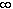#Interactive Real Analysis

Next | Previous | Glossary | Map

## 6.2. Continuous Functions

### Example 6.2.8(b):

The function f(x) = x2 is continuous on [0, 1]. Is it uniformly continuous there ?

This function (as you could guess from its graph) is uniformly continuous on the closed interval [0, 1]. To prove it, note that

| f(t) - f(s) | = | t - s | | t + s | < | t - s | 2

because s and t are in the interval [0, 1]. Hence, given any> 0 we can simply choose=/ 10 (or something similar) to prove uniform convergence. Can you fill in the details ? A similar argument, incidentally, would work on the interval [0, N] for any number N, but it would fail for the interval [0,). So, if this function then uniformly continuous on the interval [0,) ? That's the next example.

Next | Previous | Glossary | Map